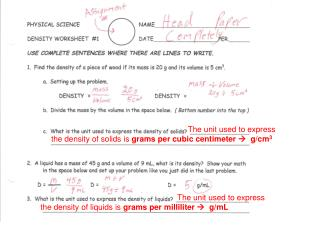# The unit used to express the density of liquids is grams per milliliter  g/mL - PowerPoint PPT PresentationDownload PresentationThe unit used to express the density of liquids is grams per milliliter  g/mL

The unit used to express the density of liquids is grams per milliliter  g/mLDownload Presentation## The unit used to express the density of liquids is grams per milliliter  g/mL

- - - - - - - - - - - - - - - - - - - - - - - - - - - E N D - - - - - - - - - - - - - - - - - - - - - - - - - - -
##### Presentation Transcript

1. The unit used to express the density of solids is grams per cubic centimeter  g/cm3 The unit used to express the density of liquids is grams per milliliter  g/mL

2. The unit used to express the density of gases is kilograms per cubic meter  kg/m3 Continue the rest of the worksheet with the same exact procedures as #4. Be sure to include all of the same steps = PRACTICE MAKES PERFECT

3. Reminder the Density Dots? Pine (wood) Air Gold Aluminum